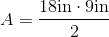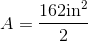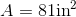# ISEE Upper Level Math : How to find the area of a kite

## Example Questions

### Example Question #1 : How To Find The Area Of A Kite

Cassie is making a kite for her little brother.  She has two plastic tubes to use as the skeleton, measuringinches andinches.  If these two tubes represent the diagnals of the kite, how many square inches of paper will she need to make the kite?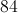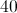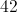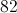Explanation:

To find the area of a kite, use the formula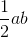, whererepresents one diagnal andrepresents the other.

Since Cassie has one tube measuringinches, we can substitutefor. We can also substitute the other tube that measuresinches in for.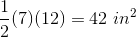### Example Question #2 : How To Find The Area Of A Kite

Two diagonals of a kite have the lengths of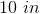and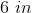. Give the area of the kite.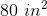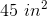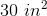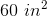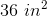Explanation:

The area of a kite is half the product of the diagonals, i.e.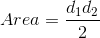,

where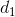and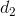are the lengths of the diagonals.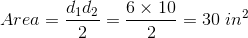### Example Question #3 : How To Find The Area Of A Kite

In the following kite,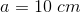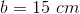and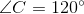. Give the area of the kite. Figure not drawn to scale.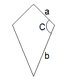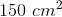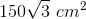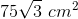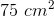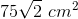Explanation:

When you know the length of two unequal sides of a kite and their included angle, the following formula can be used to find the area of a kite: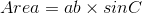,

where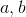are the lengths of two unequal sides,is the angle between them and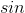is the sine function.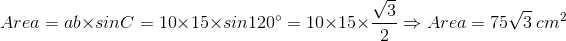### Example Question #4 : How To Find The Area Of A Kite

Find the area of a kite with one diagonal having length 18in and the other diagonal having a length that is half the first diagonal.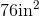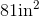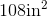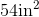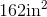Explanation:

To find the area of a kite, we will use the following formula: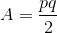where and q are the lengths of the diagonals of the kite.

Now, we know the length of one diagonal is 18in.  We also know the other diagonal is half of the first diagonal.  Therefore, the second diagonal has a length of 9in.

Knowing this, we can substitute into the formula.  We get